# tstools

### A time series toolbox for official statistics

#### 2018-02-06

The tstools package provides convenience functions to process, plot and export time series. It aims at users from fields of official statistics and macroeconomics. The package is focused on regular time series of monthly, quarterly or yearly frequency. As of February 2018, most of the functionality provided by tstools deals with plotting and so does this manual.

## Why yet another time series package?

If you have ever thought (or heard) `I can't believe it's so disgusting to create simple plots with 2 y-axes of different scales.`, or `This R thing can't do time series bar charts 'properly' - even Excel can do this. I don't get the hype.` or `Why isn't the 2010 label in the middle of the year?` tstools is for you. In other words, whenever the ‘business’ directly works with code they wonder why, e.g., legends or parts of a plot are not placed automatically where they ought to be. In addition to make interactive plotting more convenient and fun, the main goal of tstools is to provide a simple environment for production reports and plots.

Instead of claiming that the business is wrong, and placing these things automatically would limit flexibility or that the business’ visual concepts are flawed, tstools tries to provide a solution that helps economists and establishment statisticians to work and plot with R conveniently. Replace automatically with by default and you understand how tstools essentially works. The package uses R’s base plot functionality and sets a plethora of defaults, that in combination with each other make the plots look nifty. All the package does when it comes to plotting, is try to guess what the user wants when they call tsplot.

The following sections will show some examples of popular time series plots that used to be hard to get in R:

• (line) charts with 2 y-axes but matching grids
• charts with highlighted time spans
• time series bar charts with negative and positive growth contributions
• charts with a continous time x-axis

# Graphs

tstools produces base R plots. Hence all resulting plots can simply be extended by further calls to base R plot functions. Base R plots look rather technical and raw, which is why tstools tries to set a ton of useful defaults to make time series plots look fresh and clean from the start.

## Basic usage

Plotting with tstools is easy. There is only one generic plotting function called tsplot. Depending on what time series objects are passed on to the function the method dispatch chooses the right method automatically and plots the graph. The following sections will walk through several applied plotting examples. Horizontal grids that suit two axes, automatic shifting of series to the middle of the period, colors, line types, filling up started years and many other features come as convenient defaults. Defaults can be adjusted using themes.

### Single time series: line chart

The most basic example of a time series plot is a time series line chart. The snippet below loads an example dataset KOF that ships with tstools. By default year labels are placed in the middle of the year, quarters are denoted by small tick marks, years are denoted by longer ticks. In the example below, the original time series is just shortened in order to zoom in a bit. The object short is of class ts.

``````library(tstools)
data(KOF)
short <- window(KOF\$kofbarometer,start=c(2007,1),end=c(2014,1))
tsplot(short,auto_legend=F)``````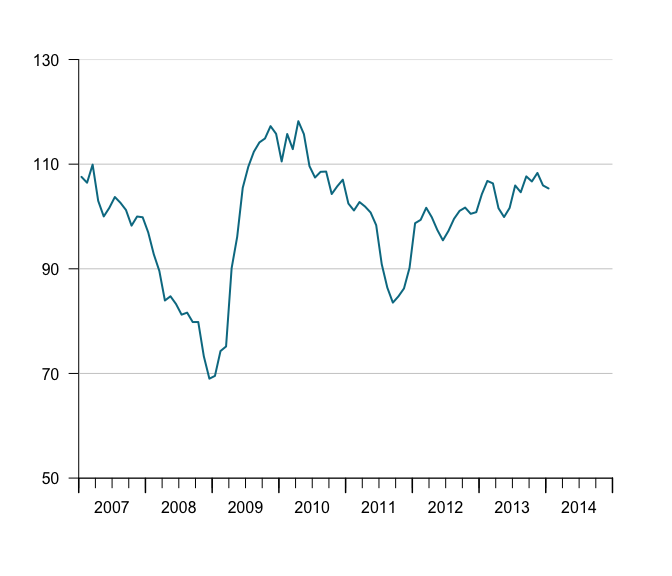### Multiple time series (same y-axis) in one line chart

The function tsplot can handle multiple time series objects or lists at once. When you want to plot several series in one plot, you can either pass multiple comma separated values to tsplot, objects of class mts or simply pass lists of time series. Consider the following example that creates a list of random time series. The series do not have be of the same frequency nor do they have to be of same length.

``````ts1 <- ts(runif(40,-10,40),start=c(1995,1),freq=4)
ts2 <- ts(runif(80,0,50),start=c(2000,1),freq=12)

tslist <- list()
tslist\$ts1 <- ts1
tslist\$ts2 <- ts2``````

Either plot single time series separated by commas:

``tsplot(ts1,ts2,auto_legend=F)``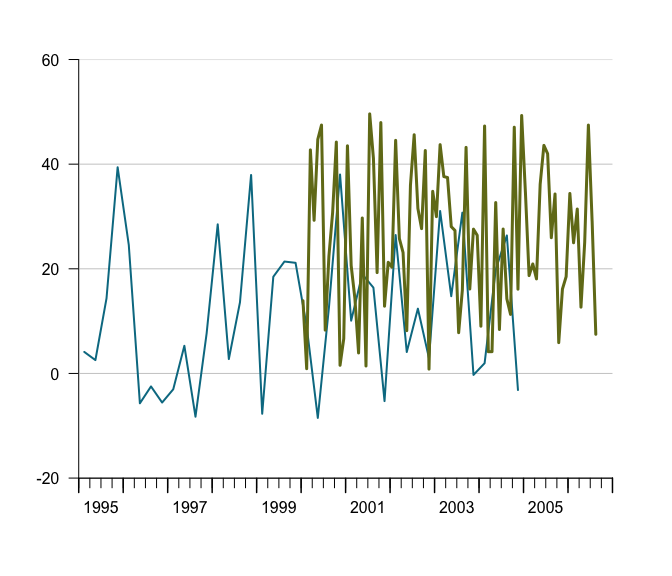or a list of time series:

``tsplot(tslist,auto_legend=F)``### Auto-scale grids are there to help you, how to configure them!

The latest release has considerably improved finding suitable scale and grids automatically. One improvement is the ability to detect not only the minimum necessary range, but also to check whether some value is so close the x-axis that we prefer one more grid for a little extra breathing room. If there’s less than 15 percent of the bottom or grid left, tsplot automatically adds another grid.

The following example tweaks this 15 percent margin to an exaggerated 30 percent, in order to show how an extra grid up top is added because of the KOF\$kofbarometer line. At the bottom no additional grid is added because all lines are further away from the x-axis than 30 percent of the grid.

``````data(KOF)
tt <- init_tsplot_theme()
tt\$y_grid_count_strict <- F
tt\$y_tick_margin <- 0.3
tsplot(KOF\$kofbarometer,
tsr = KOF\$reference,
theme = tt)``````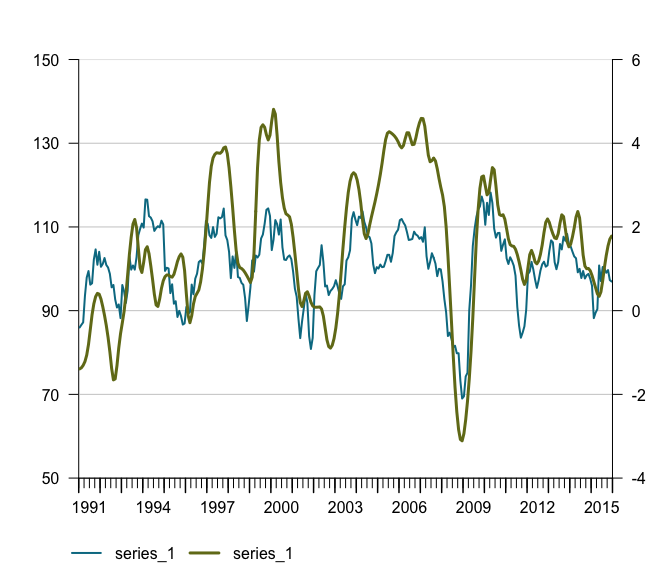### Manual value ticks

Often you just want to have a fixed scale, e.g., for an index that ranges from 0 to 100. Simply use the `manual_value_ticks_l` and `manual_value_ticks_r` arguments to specify manual ticks and grids. In case you use 2 y-axes make sure both manual value tick vectors are of the same length.

### Left Y-Axis as (stacked) bar chart

Sometimes we want to display time series as bar charts. Most plotting engines understand bar charts as something that has a categorical x-axis. So even if you have time on the x-axis, periods are treated as categories implying that a bar is centered above the category tick for that period. tstools treats the x-axis for bar charts as continous and allows a quarterly series to truly represent an entire quarter. Note that stacked bar charts imply that all involved series have the same frequency.

``````tsb1 <- ts(runif(30, -30, 20), start = c(2010, 1), frequency = 4)
tsb2 <- ts(runif(30, 0, 50), start = c(2010, 1), frequency = 4)
tsb3 <- ts(runif(30, 0, 50), start = c(2010, 1), frequency = 4)
tsplot(tsb1, tsb2, tsb3,
left_as_bar = T,
auto_legend = F)``````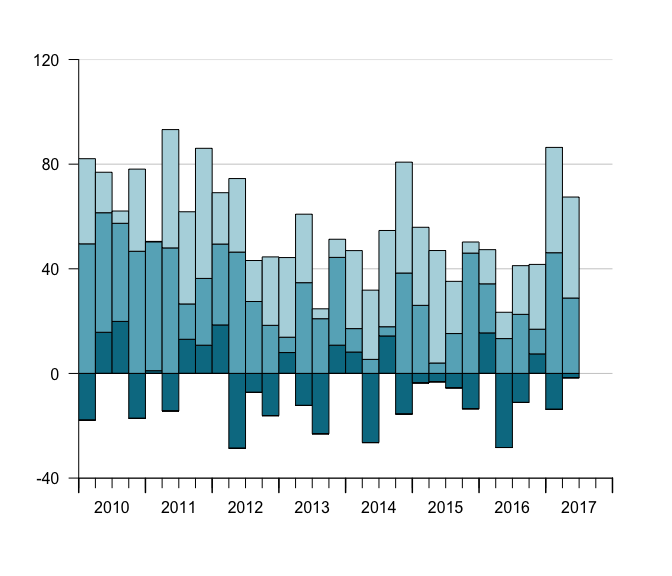### Sum as line in stacked bar charts, manual value ticks

One of the reasons for using bar charts with time series is to add up positive and negative contributions. In this case it is also helpful to be able to add the sum of the components to plot on a per period basis. The following draws a line on top of the bars that represents the sum.

``````tsl <- list(tsb1,tsb2,tsb3)
tt <- init_tsplot_theme()
tt\$sum_as_line <- T
tsplot(tsl,left_as_bar = T,
manual_value_ticks_l = seq(-40,100, by = 20),
auto_legend = F,
theme = tt)``````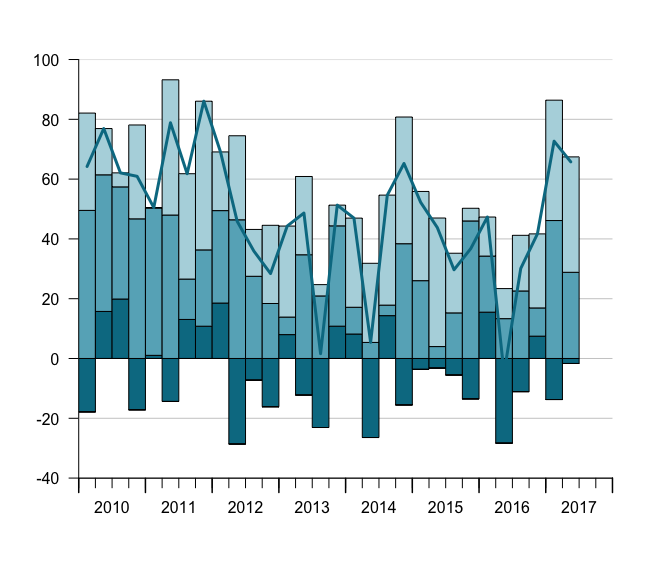Note that there’s a manual value ticks parameter in case you do not want to go with the automatically determined value ticks.

### Stacked bar charts with different start and end dates

It is even possible now, to produce stacked bar charts from time series of different start and end dates.

``````tsb1 <- ts(runif(30,-30,20),start=c(2010,1),frequency = 4)
tsb2 <- ts(runif(30,0,50),start=c(2010,1),frequency = 4)
tsb3 <- ts(runif(30,0,50),start=c(2010,1),frequency = 4)
tsb4 <- ts(runif(30,-40,10),start=c(2005,1),frequency = 4)
tsplot(tsb1, tsb2, tsb3, tsb4,
left_as_bar = T,
auto_legend = F)``````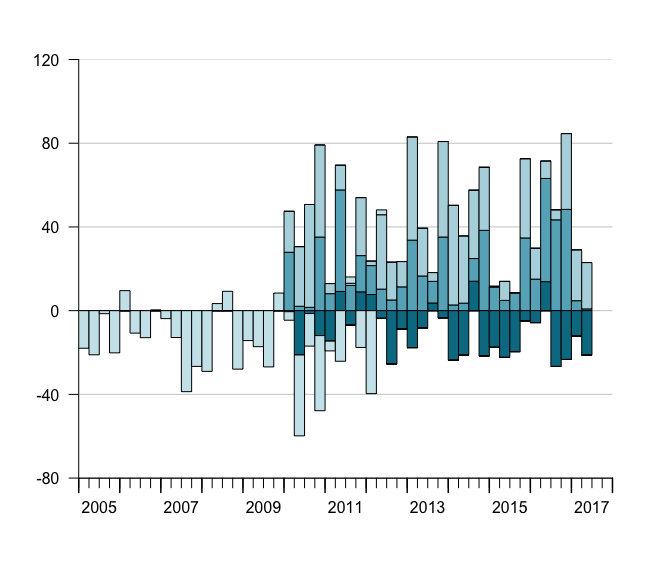### Grouped bar charts

When different variables got the same scale, but cannot be aggregated we want to display time series bars next to each other instead of stacking them. In tstools stacking is the default but it can easily be tweaked using the *group_bar_chart parameter.

``````tsb1 <- ts(runif(20,-30,20),start=c(2010,1),frequency = 12)
tsb2 <- ts(runif(20,0,50),start=c(2010,1),frequency = 12)
tsb3 <- ts(runif(20,0,50),start=c(2010,1),frequency = 12)
tsplot(tsb1, tsb2, tsb3,
left_as_bar = T,
group_bar_chart = T,
auto_legend = F)``````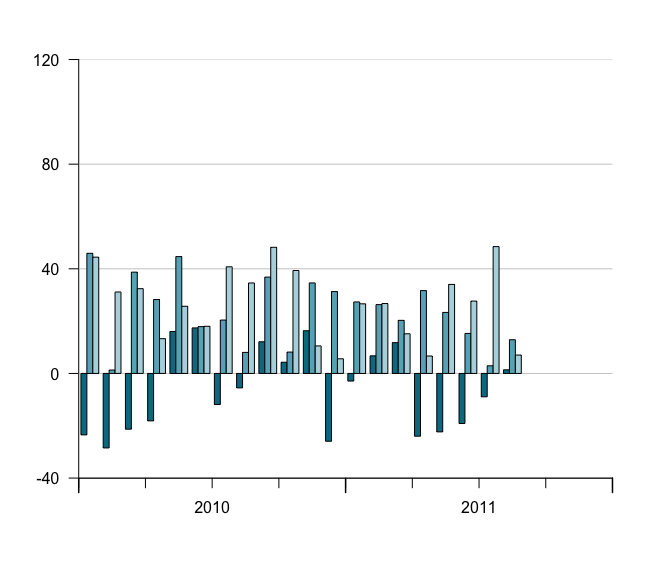### Multiple Y-axis with different scales (line charts)

In order to compare indicators it’s covenient in some domains to plot two time series of completely different scale, e.g., a growth rate and an indicator indexed at 100, to each other. Whenever the absolute level is not overly interesting but rather the lead-lag structure and the co-movement, 2 y-axes with different scales are popular. Hence tsplot introduces a second argument, tsr (time series right), which is an object of class ts or a list of time series.

``````data(KOF)
tsplot(KOF\$kofbarometer,
tsr = KOF\$reference,auto_legend=F)``````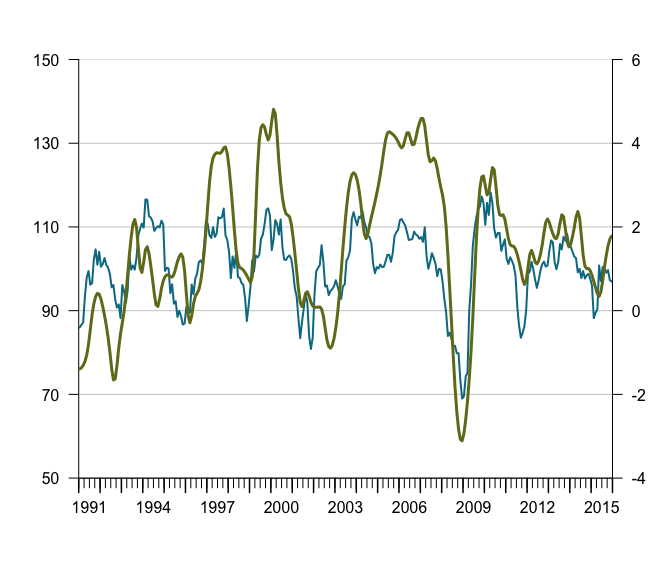### Multiple Y-axes with different scales (bar and line charts)

Sometimes you want a bar chart on one axis and an line chart on the other. Guess what, tstools also has a convenient way of creating these. Simply provide a list of time series to both the … argument and the tsr argument and choose left_as_bar = T. Note that the line chart is automatically moved to the middle of the quarterly bar.

``````tsb1 <- ts(runif(30,-30,20),start=c(2010,1),frequency = 4)
tsb2 <- ts(runif(30,0,30),start=c(2010,1),frequency = 4)
tsb3 <- ts(runif(30,0,30),start=c(2010,1),frequency = 4)
tsr1 <- ts(runif(30,-4,6),start=c(2010,1),frequency = 4)
tsplot(tsb1, tsb2, tsb3,
tsr=tsr1,
left_as_bar = T,
auto_legend=F)``````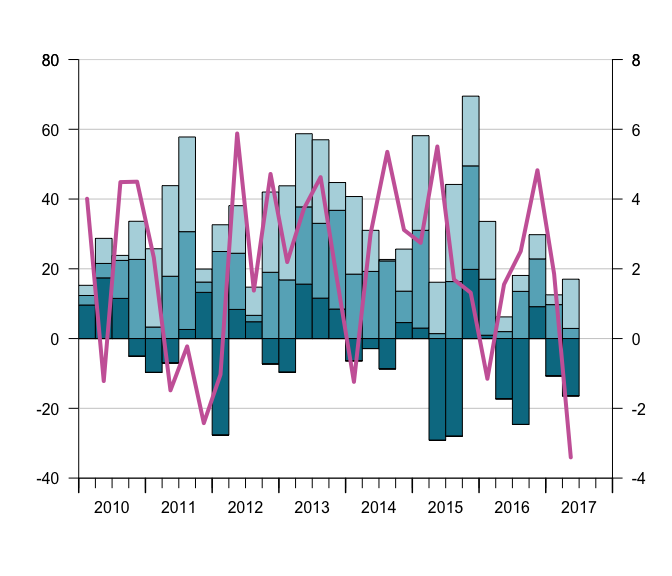## Y-Grids: automatic vs. manual

tstools tries to guess a reasonable number of ticks (and horizontal grids). This can be tricky when several time series and multiple axes are involved. tstools’ standard procedure uses value ranges and a logarithm based algorithm to find the order of magnitude of a scale. Further tstools brute forces through a number of reasonable tick counts and chooses a suitable number of ticks. In case there is more than one y-axis the choice will be passed on to the other axis.

### Using another function

However, there are countless possibilities and the number of ticks and grids may come to a matter of personal taste in the end. Hence, tstools provides not only the flexibility to set grids manually, you can even pass another algorithm implement in you very own R function that gives back a vector of ticks. Simply pass a function to the `find_ticks_function` argument. Currently range, and potential tick count are fixed as arguments to these functions, but hopefully passing other sets of arguments will be possible soon.

## Tweaking the defaults: Themes

Font size, line color, bar color, grid color, show or not show grid, and a plethora of other options would lead to a ton of parameters. If you had to specify all of those, it would be time consuming task to create a quick explorative plot. So tstools suggests many defaults to many parameters and stores these parameters in lists called themes. To tweak a default, simply initialize the default theme, tweak a single list element and pass the entire theme to tsplot. By doing so you can also define properties of multiple plots just by passing the new theme to the tsplot call.

There are way too many options to give an example for each option, but this guide will show examples of the most interesting ones. You may also want to look at `?init_tsplot_theme` or run the following to see all available options.

``````def_theme <- init_tsplot_theme()
names(def_theme)``````
``````##   "margins"                   "fillYearWithNAs"
##   "line_colors"               "line_to_middle"
##   "lwd"                       "lty"
##   "xaxs"                      "yaxs"
##   "bar_border"                "total_bar_margin_pct"
##  "bar_fill_color"            "sum_as_line"
##  "sum_line_lty"              "sum_line_lwd"
##  "sum_line_color"            "highlight_window"
##  "highlight_window_freq"     "highlight_window_start"
##  "highlight_window_end"      "highlight_color"
##  "use_box"                   "y_las"
##  "lwd_ticks_1"               "lwd_ticks_2"
##  "yearly_ticks"              "quarterly_ticks"
##  "monthly_ticks"             "tcl_quarterly_tick_tcl"
##  "tcl_yearly_tick"           "lwd_yearly_ticks"
##  "lwd_quarterly_ticks"       "label_pos"
##  "show_left_y_axis"          "show_right_y_axis"
##  "y_grid_count"              "show_y_grids"
##  "y_grid_color"              "y_grid_count_strict"
##  "y_tick_margin"             "preferred_y_gap_sizes"
##  "y_range_min_size"          "legend_col"
##  "title_line"                "title_cex.main"
##  "subtitle_outer"            "subtitle_line"
##  "subtitle_cex.main"         "subtitle_transform"
##  "legend_intersp_y"          "range_must_not_cross_zero"``````

### Highlight windows: mark a period

Consider the above graph. Let’s add a highlight window to mark the fact that 2016 and 2017 are estimates.

``````tt <- init_tsplot_theme()
tt\$highlight_window <- T
tsplot(tsb1, tsb2, tsb3,
tsr=tsr1,
left_as_bar = T,
theme = tt,
auto_legend = F)``````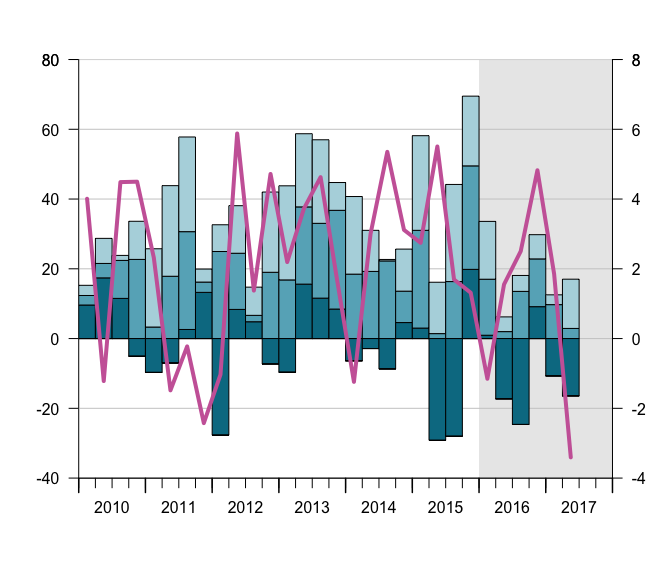### Or Get a box…

``````tt\$use_box <- T
tsplot(tsb1,tsb2,tsb3,tsr=tsr1,
left_as_bar = T,
theme = tt,
auto_legend = F)``````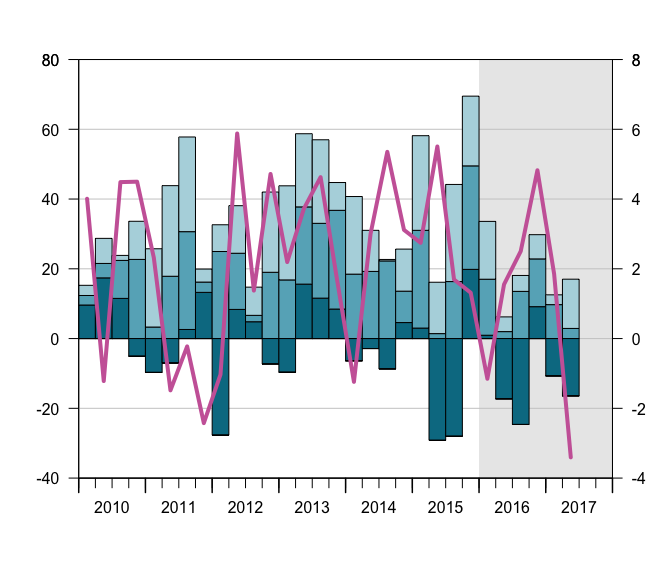### Change line types…

``````tt\$lty <- c(3,2,1)
tsplot(tsb1,tsb2,tsb3,
theme = tt,
auto_legend = F)``````